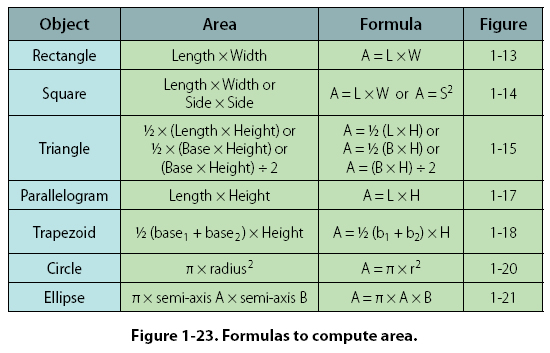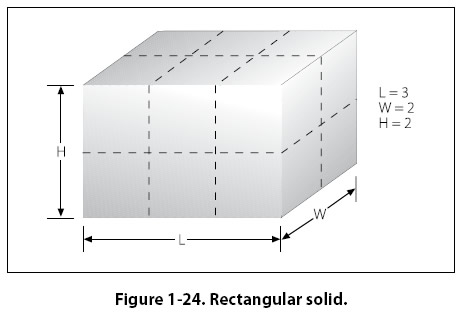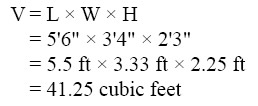Units of Area A square foot measures 1 foot by 1 foot. It also measures 12 inches by 12 inches. Therefore, one square foot also equals 144 square inches (that is, 12 x 12 = 144). To convert square feet to square inches, multiply by 144. To convert square inches to square feet, divide by 144. A square yard measures 1 yard by 1 yard. It also measures 3 feet by 3 feet. Therefore, one square yard also equals 9 square feet (that is, 3 x 3 = 9). To convert square yards to square feet, multiply by 9. To convert square feet to square yards, divide by 9. Refer to Figure 1-37, Applied Mathematics Formula Sheet, at the end of the chapter for a comparison of different units of area. Figure 1-23 summarizes the formulas for computing the area of two-dimensional solids.Computing Volume of Three-Dimensional Solids Three-dimensional solids have length, width, and height. There are many three-dimensional solids, but the most common are rectangular solids, cubes, cylinders, spheres, and cones. Volume is the amount of space within a solid. Volume is expressed in cubic units. Cubic inches or cubic centimeters are used for small spaces and cubic feet or cubic meters for larger spaces. Rectangular Solid rectangle-shaped sides. [Figure 1-24] The volume is the number of cubic units within the rectangular solid. The formula for the volume of a rectangular solid is: Volume = Length x Width x Height = L x W x HIn Figure 1-24, the rectangular solid is 3 feet by 2 feet by 2 feet. The volume of the solid in Figure 1-24 is = 3 ft x 2 ft x 2 ft = 12 cubic feet. Example: A rectangular baggage compartment measures 5 feet 6 inches in length, 3 feet 4 inches in width, and 2 feet 3 inches in height. How many cubic feet of baggage will it hold? First, substitute the known values into the formula.©AvStop Online Magazine                                                                                                                                                      Contact Us              Return To Books